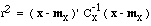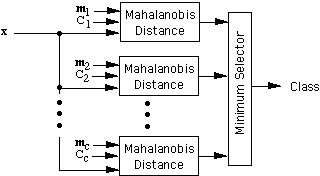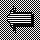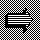# Mahalanobis Metric

The quantity r inis called the Mahalanobis distance from the feature vector x to the mean vector mx, where Cx is the covariance matrix for x. It can be shown that the surfaces on which r is constant are ellipsoids that are centered about the mean mx. In the special case where the features are uncorrelated and the variances in all directions are the same, these surfaces are spheres, and the Mahalanobis distance becomes equivalent to the Euclidean distance.

One can use the Mahalanobis distance in a minimum-distance classifier as follows. Let m1, m2, ... , mc be the means (templates) for the c classes, and let C1, C2, ... , Cc be the corresponding covariance matrices. We classify a feature vector x by measuring the Mahalanobis distance from x to each of the means, and assigning x to the class for which the Mahalanobis distance is minimum.The use of the Mahalanobis metric removes several of the limitations of the Euclidean metric:

1. It automatically accounts for the scaling of the coordinate axes

2. It corrects for correlation between the different features

3. It can provide curved as well as linear decision boundaries
However, there is a price to be paid for these advantages. The covariance matrices can be hard to determine accurately, and the memory and time requirements grow quadratically rather than linearly with the number of features. These problems may be insignificant when only a few features are needed, but they can become quite serious when the number of features becomes large.Back to MatrixOn to DiscriminantsUp to Mahalanobis